# Trigonometric Ratios and Trigonometric Identities

### Exercise 23.1

1. I have drawn a right-angled triangled ABC whose hypotenuse AB=10 cm, base BC=8 cm. and perpendicular AC=6cm. Let us determine the values of sine and tangent ABC.

2. Some have drawn a right-angled triangle ABC whose ∠ABC =90°, AB=24 cm. and BC=7cm. By calculating, let us write the values of Sin A, Cos A, tan A, and cosec A.

3. If a right-angled triangled ABC, C=90o , BC=21 units, and AB=29 units, then let us find the values of Sin A, Cos A, Sin B, and Cos B.

4. If cosØ = 7/25, then let us determine the values of all trigonometric ratios of the angle Ø.

5. If cotØ =2, then let us determine the values of tanØ and secØ and show that 1+tan2Ø=sec2Ø.

6. If cosØ=0.6, then let us show that, (5sinØ-3tanØ) =0.

7. If cotA =4/7.5, then let us determine the values of cosA and Cosec A and show that 1+cot2A=cosec2A

8. If sin C=2/3, then let us write by calculating, the value of cos C * Cosec C

### Exercise 23.2

1. In the window of our house, there is a ladder at an angle of 60o with the ground. If the ladder is 23 m. long, then let us write by calculating with the figure, the height of our window above the ground.

2. ABC is a right-angled triangle with its B is 1 right angle. If AB =83 cm. and BC =8 cm. then let us write by calculating, the values of ACB and BAC.

3. In a right-angled triangle ABC, ∠B =90o, ∠A=30o and AC =20 cm. Let us determine the length of two sides BC and AB.

4. In a right-angle triangle PQR, ∠Q=90o, ∠R=45o; if PR =32, then let us find out the lengths of two sides PQ and QR.

5. Let us determine the values of :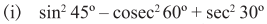5.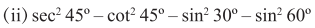5.

(iii) 3tan245o – sin2 60o – 1/3 cot230 – 1/8 sec2 45o

5.

(iv) 4/3 cot2 30o + 3sin2 60o 2 cosec 2 60o – 3/4 tan2 30o

5.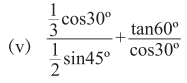5.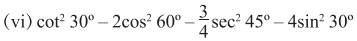5.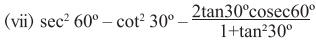5.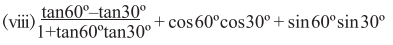5.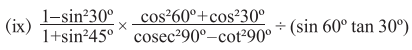6.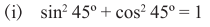6.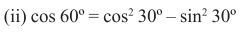6.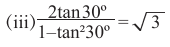6.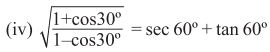6.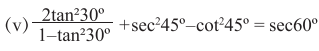6.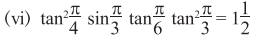6.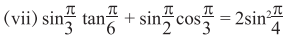7.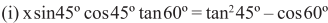, then let us determine the value of x.

7.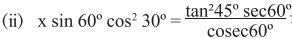then let us determine the value of x.

7.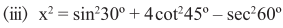then let us determine the value of x.

8. If x tan 30o + y cot 60o = 0 and 2x – y tan 45o =1, then let us write calculate the values of x and y.

9. If A=B=45o, then let us justify

(i) sin(A+B) =sinA cosB + cosA sinB

9.
(ii) cos (A+B) = cos B – sinA sinB

10. (i) In an equilateral triangle ABC, BD is a median. Let us prove that, tan ∠ABD = cot ∠BAD

10.
(ii) In an isosceles triangle ABC, AB=AC and ∠BAC=90O; the bisector of ∠BAC intersect the side BC at the point D.

11. Let us determine the value/values of Ø (0o<Ø<90o), for which 2 cos2Ø – 3cosØ + 1=0 will be true.

### Exercise 23.3

1.(i) If sinØ=4/5, then let us write the value of cosec/1+cotØ by determining it.

1.(ii) If tanØ= 3/4, then let us show that;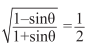1. (iii) If tanØ =1, then let us determine the value of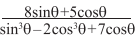2. (i) Let us express cosecØ and tanØ in terms of sinØ.
(ii) Let us write cosecØ and tanØ in terms of cosecØ.

3.(i) If secØ + tanØ =2, then let us determine the value of (secØ – tanØ).

3. (ii) If cosecØ – cotØ= √2-1, then let us write by calculating, the value of (cosecØ + cotØ).

3.(iii) If sinØ + cosØ= 1, then let us
determine the value of sinØ *cosØ.

3.(iv) If tanØ + otØ= 2, then let us determine the value of (tanØ – cotØ).

3. (v) If sinØ – cos Ø = 7/13, then let us
determine the value of sinØ + cosØ.

3(vi) If sinØcosØ=1/2, then let us write by calculating, the value of (sinØ +cosØ).

3. (vii) If secØ – tanØ = 1/√3, then let us determine the value of both secØ and tanØ.

3. (viii) If cosecØ +cotØ = √3, then let us determines the values of both cosecØ and cotØ.

3. (ix) If (sinØ+cosØ)/(sinØ – cosØ)= 7, then let us write by calculating the value of tanØ.

3.(x) If (cosecØ + sinØ)/(cosecØ – sinØ)
=5/3, then let us write by calculating, the value of sinØ.

3.(xi) If secØ + cosØ = 5/2, then let us write by calculating, the value of (secØ – cosØ).

3.(xii) Let us determine
the value of tanØ from the relation 5sin2Ø +
4cos2Ø =9/2.

3.(xiii) If tan2Ø + cot2Ø = 10/3, let us determine the value of tanØ + cotØ and tanØ – cotØ and from these let us write the value of tanØ.

3.(xiv) If sec2Ø + tan2Ø
= 13/12, then let us write by calculating, the value of (sec4Ø – tan4Ø).

4. (i) In ΔPQR, Q is right angle. If PR=√5 units and PQ-RQ=1unit, then let us determine the value of cosP – cosR.

4.(ii) In ΔXYZ, Y is right angle. If xy= 2√3 units and XZ-YZ =2
units then let us determine the value of (sec X – tan X).

5. Let us determine ‘Ø’ from the relation:

(i) x = 2sin Ø, y=3cos Ø

5.(ii) 5x= 3sec Ø, y=3tan Ø

6.(i) If sinα=5/13, then let us show that , tanα + secα =1.5.

6.(ii) If tanA = n/m, then let us determine the values of both sinA and secA.

6.(iii) If cos Ø = x/√x2+y2, then let us show that, x sin Ø = y cos Ø.

6.(iv) If sinα= a2 – b2/a2+b2.
Then let us show that , cot α =2ab/a2 – b2.

6.(v) If sin Ø/x = cos Ø/y, then let us show that, sin Ø – soc Ø = x-y/√x2+y2.

6.(vi) If (1+4x2)  cosA = 4x, then let us show that cosecA +
cotA = 1+2x/1-2x.

7. If x = a sin Ø and y= b tan Ø, then let us prove that, a2/x2 – b2/y2=1.

8.If sin Ø + sin2 = 1, then let us prove that , cos2 Ø + cos4 Ø = 1.

## Related Posts

### Right Circular Cone

Q.1 I have made a closed right circular cone whose length of the base radius is 15 cm and slant height is 24 cm. Let

### Trigonometric Ratios of Complementary Angle

1. Let us evaluate:                                  2. Let us show that:

### Rectangular Parallelopiped or Cuboid

3. The length, breadth, and height of a cuboidal Room are 5 m, 4 m, and 3m respectively, let us write the length of the

### Trigonometry : Concept of Measurement of Angle

Exercise 20 Let us express the following into degrees, minutes, and seconds. (i) 832’     (ii) 6312”    (iii) 375”(iv) 27 (1/12)o    (v) 72.04o